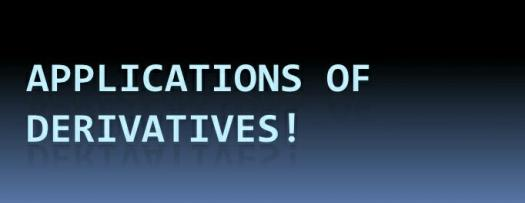# Applications Of Derivatives Assessment Test

10 Questions | Total Attempts: 111SettingsTake our intelligent assessment test to assess your knowledge of derivative application, which is the method used in our everyday life such as determining concavity, curve sketching and optimization.

Related Topics
• 1.
The process of finding a derivative is called...
• A.

Calculation

• B.

Slope

• C.

Working

• D.

Differentiation

• 2.
The derivative of a function of a real variable measures the sensitivity to change of the function value...
• A.

True

• B.

False

• C.

Probably

• D.

Maybe

• 3.
The derivative of the position of a moving object with respect to time is the object's...
• A.

Time

• B.

Distance

• C.

Speed

• D.

Velocity

• 4.
The matrix that represents this linear transformation with respect to the basis given by the choice of independent and dependent variables is the...
• A.

Angle

• B.

Tangent

• C.

Jacobian matrix

• D.

Linear matrix

• 5.
The ratio of the instantaneous change in the dependent variable to that of the independent variable is termed the...
• A.

Slope

• B.

Tanvrnt

• C.

Derivative

• D.

Line

• 6.
The derivative of a second derivative is called...
• A.

Product

• B.

Third derivative

• C.

Derived

• D.

Deriver

• 7.
A function that has infinitely many derivatives is called infinitely differentiable or...
• A.

Smooth

• B.

Hard

• C.

High

• D.

Low

• 8.
On the real line, every polynomial function is infinitely differentiable.
• A.

True

• B.

False

• C.

Maybe

• D.

Depends on some factors

• 9.
If a polynomial of degree n is differentiated n times, then it becomes...
• A.

Grip function

• B.

Constant function

• C.

Slope

• D.

Derivative

• 10.
A point where the second derivative of a function changes sign is called...
• A.

Inflection point

• B.

Slope

• C.

Joint

• D.

Curve